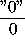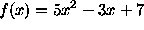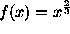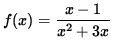### DERIVATIVES USING THE LIMIT DEFINITION

The following problems require the use of the limit definition of a derivative, which is given by.

They range in difficulty from easy to somewhat challenging. If you are going to try these problems before looking at the solutions, you can avoid common mistakes by making proper use of functional notation and careful use of basic algebra. Keep in mind that the goal (in most cases) of these types of problems is to be able to divide out theterm so that the indeterminant formof the expression can be circumvented and the limit can be calculated.

• PROBLEM 1 : Use the limit definition to compute the derivative, f'(x), for.

• PROBLEM 2 : Use the limit definition to compute the derivative, f'(x), for.

• PROBLEM 3 : Use the limit definition to compute the derivative, f'(x), for.

• PROBLEM 4 : Use the limit definition to compute the derivative, f'(x), for.

• PROBLEM 5 : Use the limit definition to compute the derivative, f'(x), for.

This problem may be more difficult than it first appears.

• PROBLEM 6 : Use the limit definition to compute the derivative, f'(x), for.

• PROBLEM 7 : Use the limit definition to compute the derivative, f'(x), for.

• PROBLEM 8 : Use the limit definition to compute the derivative, f'(x), for.

• PROBLEM 9 : Assume thatShow that f is differentiable at x=1, i.e., use the limit definition of the derivative to compute f'(1) .

• PROBLEM 10 : Assume thatShow that f is differentiable at x=0, i.e., use the limit definition of the derivative to compute f'(0) .

• PROBLEM 11 : Use the limit definition to compute the derivative, f'(x), for

f(x) = | x2 - 3x | .

• PROBLEM 12 : Assume thatDetermine if f is differentiable at x=2, i.e., determine if f'(2) exists.